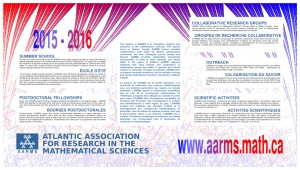## Our 2015 – 2016 PosterThe image shows a fractal set (called “Christiane’s Hair” in a recent paper) that is constructed by vertically “stacking” Cantor sets with continuously changing scaling ratios. The binary tree structure of the Cantor set is thereby emphasized, with “gaps’’ at a certain size scale separating the branches of the binary tree at that size scale. The construction also highlights the fact that the Cantor set is constructed by “ripping apart” the interval and inserting gaps. While each horizontal slice of the image is self-similar, the entire set is not since the scaling ratios vary. The image is colored in a self-similar manner, according to the percentage of 0’s and 1’s in the “address” of the given point (the proportion of left or right braches taken in the traversing the binary tree).

Fractals are mathematical objects that exhibit some type of scaling behaviour. The most commonly encountered (and popular) fractals are geometric sets, although there exist a very large variety of fractal constructions. Fractals have been studied within “pure” mathematics as examples of objects with very interesting behaviour (for example, see below for some properties of the Cantor set). Fractals have also been useful in many areas of applied science, in characterizing or describing complicated phenomena. Fractals can be obtained as the supports of invariant measures of iterated function systems (IFS) with probabilities. Invariant measures also describe long term statistical behaviour of orbits of iterated function systems.

The Cantor (ternary) set is perhaps the oldest known example of a fractal set and is commonly used as an example of a set with somewhat startling properties. One way to obtain the Cantor set is to start with a closed interval of unit length, and remove the middle third leaving two scaled down closed intervals each with length equal to one-third. Then these closed intervals have their middle thirds removed, and the process is repeated ad infinitum. The Cantor set is closed and compact. It is dense-in-itself since every open set containing a point from the Cantor Set contains other points from the Cantor set. Thus it is not scattered. However, it is totally disconnected: given any two points in the Cantor set, there exist two open sets in the plane that are disjoint and each set contains one of the given points. The Cantor set is uncountable, despite a geometrical way to obtain it that involves countably many steps. There is no length left after removing all the middle thirds. The scaling dimension is ln 2/ln 3, approximately 0.63. This dimension between 0 and 1 is consistent with the fact that there are too many points to count, but no length to measure.

– Viqar Husain